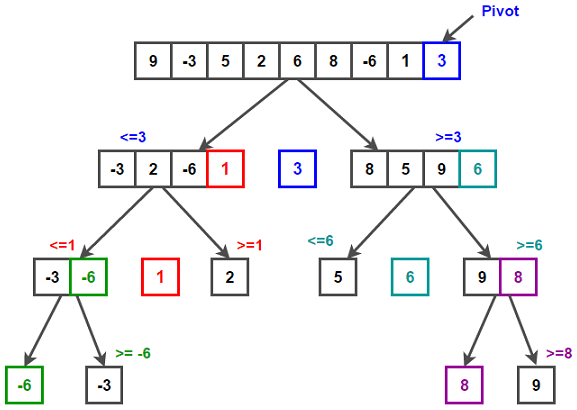# Quicksort Algorithm

Given an array of integers, sort it using quicksort algorithm.

Quicksort is an efficient in-place sorting algorithm, which usually performs about two to three times faster than merge sort and heapsort when implemented well. Quicksort is a comparison sort, meaning that it can sort items of any type for which a less-than relation is defined. In efficient implementations, it is usually not a stable sort.

Quicksort on average takes O(nlog(n)) comparisons to sort n items. In the worst case, it makes O(n2) comparisons, though this behavior is very rare.

## How Quicksort works?

Quicksort is a divide and conquer algorithm. Like all divide and conquer algorithms, it first divides a large array into two smaller sub-arrays and then recursively sort the sub-arrays. Basically, three steps are involved in whole process –

• Pivot selection: Pick an element, called a pivot, from the array (usually the leftmost or the rightmost element of the partition).

• Partitioning: Reorder the array so that all elements with values less than the pivot come before the pivot, while all elements with values greater than the pivot come after it (equal values can go either way). After this partitioning, the pivot is in its final position.

• Recurse: Recursively apply the above steps to the sub-array of elements with smaller values than pivot and separately to the sub-array of elements with greater values than pivot.

The base case of the recursion is arrays of size 1, which never need to be sorted.

Below diagram shows how at each step of the quicksort algorithm we choose leftmost element as pivot, partition the array across pivot and recurse on two sub-arrays we get after partition process.Please note that the pivot selection and partitioning steps can be done in several different ways and the choice of specific implementation schemes greatly affects the algorithm’s performance. We will discuss all that in detail later, but let’s now get to the coding part.

Below is C++ and Java implementation of quicksort algorithm –

## Java

Output:

-6 -3 1 2 3 5 6 8 9

## Quicksort Performance:

The worst case time complexity of Quicksort is O(n2). The worst case happens when the pivot happens to be the smallest or largest element in the list, or when all the elements of array are equal. This will result in most unbalanced partition as the pivot divides the array into two sub-array of sizes 0 and n – 1. If this happens repeatedly in every partition (example, we have sorted array), then each recursive call processes a list of size one less than the previous list and that will result in O(n²) time.

T(n) = T(n-1) + cn = O(n²)

(Note – partition takes O(n) time that accounts for cn)

The best case time complexity of quicksort is O(nlog(n)). The best case happens when the pivot divides the array into two nearly equal pieces. Now each recursive call processes a list of half the size.

T(n) = 2 T(n/2) + cn = O(n log(n))

Must Read: How to Boost Quicksort performance?

References: https://en.wikipedia.org/wiki/Quicksort(4 votes, average: 5.00 out of 5)Loading...

Please use our online compiler to post code in comments. To contribute, get in touch with us.
Like us? Please spread the word and help us grow. Happy coding 🙂Subscribe
Notify ofGuest

Quicksort is a beautiful algorithm..Guest